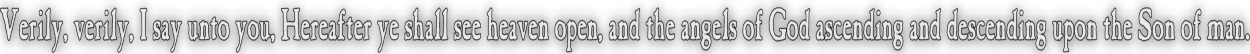None:
Polyps:
Strongs:CreationismThe Way Of The Kings Of The East Rev 16:12 And the sixth angel poured out his vial upon the great river Euphrates; and the water thereof was dried up, that the way of the kings of the east might be prepared. (KJV) Given our "Sun octal" - [0,a,b,c,d,e,f,g] under a seven cycle (a,b,d,c,f,g,e) we may write out the seven cycle on the subgroups of the octal in columns as so; a = 1 = [b,d,f] b = [c,d,g] d = [c,e,f] c = [a,f,g] f = [b,e,g] g = [a,d,e] e = [a,b,c] When we came to the trumpets and the resurrection of two witnesses we wrote out the left hand octal in a column on the right, thus; [b,d,f] = 1 = a = {c,e,g} [c,d,g] = b = {a,e,f} [c,e,f] = d = {a,b,g} [a,f,g] = c = {b,d,e} [b,e,g] = f = {a,c,d} [a,d,e] = g = {b,c,f} [a,b,c] = e = {d,f,g} The viols of wrath poured out on the octal sea press every living person into spiritually dwelling in the four earthy elements of the original "Sun" octal. The picture of the believer is to be dwelling in heavenly places with the Lord - stood upon a "Sea of glass". The Lord is as GF(8) with GF(4) transformed by it, and since every octal and choice of seven cycle and unity are essentially isomorphic, when the "Euphrates" octal is dried up we may no longer write second or third generation octals and we are restricted to the one left/right pair. Essentially every octal is aligned in isomorphism to the one reference octal (in the variable "x") as follows; [b,d,f] = 1 = a = {c,e,g} = 1 [c,d,g] = b = {a,e,f} = x [c,e,f] = d = {a,b,g} = x2 [a,f,g] = c = {b,d,e} = x+1 = x3 [b,e,g] = f = {a,c,d} = x2 + x = x4 [a,d,e] = g = {b,c,f} = x2 + x + 1 = x5 [a,b,c] = e = {d,f,g} = x2 + 1 = x6 Now, there is only one real analogy to the kings of the east in the new testament - that visit of those three sages who brought the gifts of gold, frankincense and myrrh to Christ at his birth. Whilst they are not "kings", some astrologers would have plausibly been attached to a noble and would have been dispatched from said same king or court in which they served their own lord's as astrologers or wise men. Then it is apparent that the Christ and the star over His birthplace would have been acknowledged abroad and the sages were dispatched out of reverence - (rather than for a foreign king to move court into Judea, unthinkable) - these wise men were as philosophical ambassadors for what were in that day at least, the kings of the east. By figure we would place Christ and the dwelling place in sabbath rest of His sealed (as unity) over the earth and the path of the kings from east to west under the heavens (And sacrifice of the lamb as unity before the throne) with the earth aligned to the bottom four rows in every octal. So if every octal is aligned with the static subgroup at the top and lastly the four earthy elements below, then side by side they would align to the one isomorphic finite field in polynomials as thus; x = ... = b x2 = ... = d x4 = ... = f 1 = ... = 1 = a x3 = ... = c x5 = ... = g x6 = ... = e And we appropriately see the world divided between Jesus Chris's left and His right, heavenly and earthy respectively. Note the identity or "morning star" or "Christ with two parts cut off" - the lamb as offered on the altar before the throne (unity) is in the four earthy elements but above the three remaining earthy elements. Also note that if we wrote this out as usual the polynomials would be on the right and the right hand octal as usual in the leftmost column. However, if every such octal is written out with the sun octal on the right as a writer of Hebrew would do so, then we move to the representation in polynomials (the generalised case) from right to left (As Hebrew is written right to left) so that the path of the (three) "kings" is from right to left - i.e. from the "east". - as if the three kings (the remaining earthy elements {c,g,e} above) follow the star (unity). Since every such octal field aligns as such, the way of the kings from the east is prepared so that the whole of the earth will gravitate into the one representation below the sea of glass or GF(8). The Lord's enemies become His footstool. We may align not just 30 octals in the same seven symbols, but any octal group - all are isomorphic.(I do not refer to other groups with 8 elements) This way of the kings of the east being prepared or opened up is the action of the winepress of God's wrath, which elsewhere is not specifically described but is deduced from the viols poured out as they are in sequence, forcing the inhabitants of the sea into the four earthy elements in the one octal to the Lord's right. This becomes the basis for the expectation of the return of Christ and the containment in the lake of fire of the beast and false prophet. Continue To Next Page Return To Section Start Return To Previous Page'